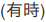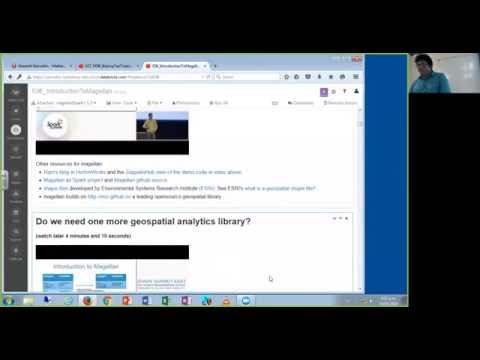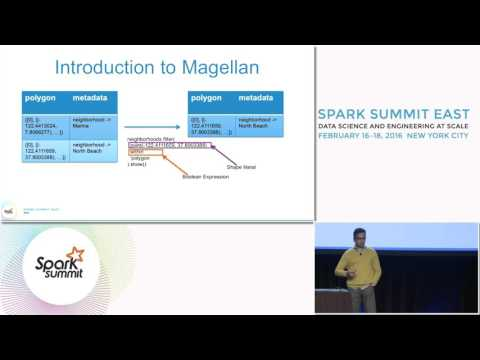// Databricks notebook source exported at Tue, 28 Jun 2016 09:59:03 UTC

# Scalable Data Science

### prepared by Dillon George, Raazesh Sainudiin and Sivanand Sivaram

The html source url of this databricks notebook and its recorded Uji:# Introduction to Magellan for Scalable Geospatial Analytics

This is a minor augmentation of Ram Harsha’s Magellan code blogged here:

First you need to attach the following two libraries:

See using 3rd party libraries in databricks for help on attaching libraries in databricks.

## Magellan-Spark as a Scalable Geospatial Analytics Engine

HOMEWORK: Watch the magellan presentation by Ram Harsha (Hortonworks) in Spark Summit East 2016.

![Ram harsha’s Magellan Spark Summit East 2016 Talk]](https://www.youtube.com/watch?v=1lF1oSjxMT4)

Other resources for magellan:

## Do we need one more geospatial analytics library?

(watch later 4 minutes and 10 seconds):• Spatial Analytics at scale is challenging
• Simplicity + Scalability = Hard
• Ancient Data Formats
• metadata, indexing not handled well, inefficient storage
• Geospatial Analytics is not simply Business Intelligence anymore
• Statistical + Machine Learning being leveraged in geospatial
• Now is the time to do it!
• Explosion of mobile data
• Finer granularity of data collection for geometries
• Analytics stretching the limits of traditional approaches
• Spark SQL + Catalyst + Tungsten makes extensible SQL engines easier than ever before!

## Nuts and Bolts of Magellan

Let us first import what we will need for our geo-spatial analysis below.


// import statements are below NOTE: this magellan needs spark 1.5.1 and the jar was built from source and attached as a snapshot here
import magellan.{Point, Polygon, PolyLine}
import org.apache.spark.sql.magellan.MagellanContext
import org.apache.spark.sql.magellan.dsl.expressions._
import org.apache.spark.sql.Row
import org.apache.spark.sql.types._
import org.apache.spark.sql.functions._ // this is needed for sql functions like explode, etc.

import java.sql.Timestamp
import java.text.SimpleDateFormat



### Data Structure: Point


val points = sc.parallelize(Seq((-1.0, -1.0), (-1.0, 1.0), (1.0, -1.0)))
.toDF("x", "y")
.select(point($"x",$"y").as("point"))



points.show(false)



### Data Structure: Polygon


case class PolygonRecord(polygon: Polygon) // let's create a case class for magellan polygon

val ring = Array(new Point(1.0, 1.0), new Point(1.0, -1.0),
new Point(-1.0, -1.0), new Point(-1.0, 1.0),
new Point(1.0, 1.0))



The val ring above encode the square polygon given by $$[-1,1]^2$$.


// let's creat a data frame of polygons
val polygons = sc.parallelize(Seq(
PolygonRecord(new Polygon(Array(0), ring))
)).toDF()



polygons.show(false)



### Predicate: within


polygons.select(point(0.5, 0.5) within $"polygon").show(false) //.count()  ### Predicate: intersects The join leverages SparkSQL’s Catalyst and Tungsten.  points.join(polygons).show(false)   points.join(polygons).where($"point" intersects $"polygon").show(false) // all these points intersect the polygon  Let’s add another point $$(-2,-2)$$ that lies outside our $$[-1,1]^2$$ polygon.  val morePoints = sc.parallelize(Seq((-1.0, -1.0), (-1.0, 1.0), (1.0, -1.0), (-2.0,-2.0))) .toDF("x", "y") .select(point($"x", $"y").as("point"))   morePoints.join(polygons).show(false)   morePoints.join(polygons).where($"point" intersects $"polygon").show(false) // (-2,-2) is not in the polygon  ## Uber Dataset for the Demo done by Ram Harsha in Europe Spark Summit 2015 First the datasets have to be loaded. See the section below on Downloading datasets and putting them in distributed file system for doing this anew (This only needs to be done once if the data is persisted in the distributed file system). After downloading the data, we expect to have the following files in distributed file system (dbfs): • all.tsv is the file of all uber trajectories • SFNbhd is the directory containing SF neighborhood shape files.  display(dbutils.fs.ls("dbfs:/datasets/magellan/")) // display the contents of the dbfs directory "dbfs:/datasets/magellan/"  First five lines or rows of the uber data containing: tripID, timestamp, Lon, Lat  sc.textFile("dbfs:/datasets/magellan/all.tsv").take(5).foreach(println)   display(dbutils.fs.ls("dbfs:/datasets/magellan/SFNbhd")) // legacy shape files  #### Homework First watch the more technical magellan presentation by Ram Sri Harsha (Hortonworks) in Spark Summit Europe 2015 ![Ram Sri Harsha’s Magellan Spark Summit EU 2015 Talk]](https://www.youtube.com/watch?v=rP8H-xQTuM0) Second, carefully repeat Ram’s original analysis from the following blog as done below.  case class UberRecord(tripId: String, timestamp: String, point: Point) // a case class for UberRecord   val uber = sc.textFile("dbfs:/datasets/magellan/all.tsv") .map { line => val parts = line.split("\t" ) val tripId = parts(0) val timestamp = parts(1) val point = Point(parts(3).toDouble, parts(2).toDouble) UberRecord(tripId, timestamp, point) }.repartition(100) .toDF() .cache()   val uberRecordCount = uber.count() // how many Uber records?  So there are over a million UberRecords.  val neighborhoods = sqlContext.read.format("magellan") .load("dbfs:/datasets/magellan/SFNbhd/") .select($"polygon", $"metadata") .cache()   neighborhoods.count() // how many neighbourhoods in SF?   neighborhoods.printSchema   neighborhoods.take(2) // see the first two neighbourhoods   neighborhoods.select(explode($"metadata").as(Seq("k", "v"))).show(5,false)



This join yields nothing.

So what’s going on?

Watch Ram’s 2015 Spark Summit talk for details on geospatial formats and transformations.


neighborhoods
.join(uber)
.where($"point" within$"polygon")
.select($"tripId",$"timestamp", explode($"metadata").as(Seq("k", "v"))) .withColumnRenamed("v", "neighborhood") .drop("k") .show(5)  Need the right transformer to transform the points into the right coordinate system of the shape files.  val transformer: Point => Point = (point: Point) => { val from = new NAD83(Map("zone" -> 403)).from() val p = point.transform(from) new Point(3.28084 * p.x, 3.28084 * p.y) } // add a new column in nad83 coordinates val uberTransformed = uber .withColumn("nad83",$"point".transform(transformer))
.cache()



Let’ try the join again after appropriate transformation of coordinate system.


val joined = neighborhoods
.join(uberTransformed)
.where($"nad83" within$"polygon")
.select($"tripId",$"timestamp", explode($"metadata").as(Seq("k", "v"))) .withColumnRenamed("v", "neighborhood") .drop("k") .cache()   joined.show(5,false)   val UberRecordsInNbhdsCount = joined.count() // about 131 seconds for first action (doing broadcast hash join)   uberRecordCount - UberRecordsInNbhdsCount // records not in the neighbouthood shape files   joined .groupBy($"neighborhood")
.agg(countDistinct("tripId")
.as("trips"))
.orderBy(col("trips").desc)
.show(5,false)



## Spatio-temporal Queries

can be expressed in SQL using the Boolean predicates such as, $$\in , \cap, \ldots$$, that operate over space-time sets given products of 2D magellan objects and 1D time intervals.

Want to scalably do the following:

• Given :
• a set of trajectories as labelled points in space-time and
• a product of a time interval [ts,te] and a polygon P
• Find all labelled space-time points that satisfy the following relations:
• intersect with [ts,te] X P
• the start-time of the ride or the end time of the ride intersects with [ts,te] X P
• intersect within a given distance d of any point or a given point in P (optional)

This will allow us to answer questions like:

• Where did the passengers who were using Uber and present in the SoMa neighbourhood in a given time interval get off?

## Other spatial Algorithms in Spark are being explored for generic and more efficient scalable geospatial analytic tasks

See the Spark Summit East 2016 Talk by Ram on “what next?”

• SpatialSpark aims to provide efficient spatial operations using Apache Spark.
• Spatial Partition
• Generate a spatial partition from input dataset, currently Fixed-Grid Partition (FGP), Binary-Split Partition (BSP) and Sort-Tile Partition (STP) are supported.
• Spatial Range Query
• includes both indexed and non-indexed query (useful for neighbourhood searches)
• z-order Knn join
• A space-filling curve trick to index multi-dimensional metric data into 1 Dimension. See: ieee paper and the slides.
• AkNN = All K Nearest Neighbours - identify the k nearesy neighbours for all nodes simultaneously (cont AkNN is the streaming form of AkNN)
• need to identify the right resources to do this scalably.
• spark-knn-graphs: https://github.com/tdebatty/spark-knn-graphs ** **

## getting uber data

### (This only needs to be done once per shard!)


%sh ls



%sh
wget https://raw.githubusercontent.com/dima42/uber-gps-analysis/master/gpsdata/all.tsv



%sh
pwd



dbutils.fs.mkdirs("dbfs:/datasets/magellan") //need not be done again!



dbutils.fs.cp("file:/databricks/driver/all.tsv", "dbfs:/datasets/magellan/")



## Getting SF Neighborhood Data


%sh
wget http://www.math.canterbury.ac.nz/~r.sainudiin/courses/ScalableDataScience/2016/datasets/magellan/UberSF/planning_neighborhoods.zip



%sh
unzip planning_neighborhoods.zip



%sh
mv planning_neighborhoods.zip orig_planning_neighborhoods.zip



%sh
mkdir SFNbhd && mv planning_nei* SFNbhd && ls
ls SFNbhd



dbutils.fs.mkdirs("dbfs:/datasets/magellan/SFNbhd") //need not be done again!



dbutils.fs.cp("file:/databricks/driver/SFNbhd/planning_neighborhoods.dbf", "dbfs:/datasets/magellan/SFNbhd/")
dbutils.fs.cp("file:/databricks/driver/SFNbhd/planning_neighborhoods.prj", "dbfs:/datasets/magellan/SFNbhd/")
dbutils.fs.cp("file:/databricks/driver/SFNbhd/planning_neighborhoods.sbn", "dbfs:/datasets/magellan/SFNbhd/")
dbutils.fs.cp("file:/databricks/driver/SFNbhd/planning_neighborhoods.sbx", "dbfs:/datasets/magellan/SFNbhd/")
dbutils.fs.cp("file:/databricks/driver/SFNbhd/planning_neighborhoods.shp", "dbfs:/datasets/magellan/SFNbhd/")
dbutils.fs.cp("file:/databricks/driver/SFNbhd/planning_neighborhoods.shp.xml", "dbfs:/datasets/magellan/SFNbhd/")
dbutils.fs.cp("file:/databricks/driver/SFNbhd/planning_neighborhoods.shx", "dbfs:/datasets/magellan/SFNbhd/")



display(dbutils.fs.ls("dbfs:/datasets/magellan/SFNbhd/"))